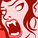# QlikView App Dev

Discussion Board for collaboration related to QlikView App Development.

cancel
Showing results for
Did you mean:Creator

## Data Labels adjustment on multiple line chart

Hi All,

I am making a dashboard where in i have combo chart that has bar and multiple lines.

now the problem is the numbers on line are overlapping. Is there any way i can display data labels for one line above it and data labels for other line below it.

I know how to do it but for numbers only for simple expression but here i have below expressions :

expr 1:

=num((\$(Vol)-\$(Delay))/(\$(Vol)),'##.00')

\$(Vol) = sum(P_Volume)

\$(Delay) = count({\$<[P_Delay(Y/N)]={'Y'}>}[P_Delay(Y/N)])

expr 2:

=((sum([P_QC Count])-count({\$<[P_Internal Error]={">=1"}>}[P_Internal Error])))/sum([P_QC Count])

Can anyone suggest any way by which i can achieve data labels adjustment here also.

Note: i can not change chart type.

Regards

SagarMVP

You can try this?

Exp 1:

Dual(Chr(10) & num((\$(Vol)-\$(Delay))/(\$(Vol)),'##.00')

\$(Vol) = sum(P_Volume)

\$(Delay) = count({\$<[P_Delay(Y/N)]={'Y'}>}[P_Delay(Y/N)]), num((\$(Vol)-\$(Delay))/(\$(Vol)),'##.00')

\$(Vol) = sum(P_Volume)

\$(Delay) = count({\$<[P_Delay(Y/N)]={'Y'}>}[P_Delay(Y/N)]))

Exp 2:

Dual(Chr(10) & ((sum([P_QC Count])-count({\$<[P_Internal Error]={">=1"}>}[P_Internal Error])))/sum([P_QC Count]),

((sum([P_QC Count])-count({\$<[P_Internal Error]={">=1"}>}[P_Internal Error])))/sum([P_QC Count])

)

Note - If this issue not resolved, I suggest you to share sample?

Before develop something, think If placed (The Right information | To the right people | At the Right time | In the Right place | With the Right context)